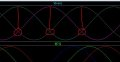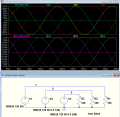# Three phase symetrical system (zero current on the nutral). Where are the currents go???

#### babaliaris

Joined Nov 19, 2019
158
This is the first time in circuits that something doesn't make sense. In all the circuits that I've learned so far, the same amount of current that is drawn from the source returns back to the minus terminal of the source. According to our professors, this is a key characteristic, in order for Kirchhoff's laws to stand.

This does not seem to be happening in a symmetrical three-phase (Y-Y configuration) circuit. All 3 currents start at the positive terminals of each source and then through each line they enter each load and end up on the neutral. But the neutral current is zero. Where do the currents go from there? Do they sum up somehow, maybe exterminating each other (that's unlikely)? In a Delta configuration, things are more confusing...

My tutor told me I will understand when I'll learn Electromagnetic Theory, but thinking in a Kirchhoff way, why it doesn't make sense? Once all the currents in the load reach the neutral (I1+I2+I3 = 0), how are they returning back to the sources?

Thank you.

#### BobTPH

Joined Jun 5, 2013
6,518
When one phase is at it’s peak what is the voltage on the other two phases?

Bob

•drjohsmith and babaliaris

#### Ian0

Joined Aug 7, 2020
7,459
What is the average live voltage? (L1+L2+L3)/3

It is therefore any surprise that the neutral current is zero?

•babaliaris

#### babaliaris

Joined Nov 19, 2019
158
When one phase is at it’s peak what is the voltage on the other two phases?

Bob
They seem to cross each other:Does this mean that they are equal to each other? Which means if I bring one of them on the other side of the equation, the sum becomes zero? Which means the phase that is at its peak value is the only one conducting at that point in time?

Joined Jul 18, 2013
27,023
This is very similar to a question posed in a 1st year N.A. electricians test.
In N.A. the residential supply is a 1ph transformer with a centre tapped secondary 120vac-0v-120vac. (L1-N-L2)
Outlets are 120v 15a rated.
If a three conductor cable is ran to dual socket outlets with L1 & N feeding one outlet and L2 and N on the other outlet (using the shared neutral) if a load of 120v 10a is plugged into the top outlet and a load of 120v 10amp load on the bottom outlet, what is the current in the common-shared neutral?

#### schmitt trigger

Joined Jul 12, 2010
588
To understand three phase current, you have to understand vectors. And how to add them.
In a BALANCED three phase wye system, the neutral’s vector sum is zero.

•Delta Prime and ericgibbs

#### nsaspook

Joined Aug 27, 2009
11,278
They seem to cross each other:
View attachment 262082

Does this mean that they are equal to each other? Which means if I bring one of them on the other side of the equation, the sum becomes zero? Which means the phase that is at its peak value is the only one conducting at that point in time?
Three phase power is a rotating field so you need to think in 3D vector rotation and time to see how the summation of the currents results into zero current at the star or neutral point.
https://www.technocrazed.com/9-5-three-phase-y-and-delta-configurations

#### BobTPH

Joined Jun 5, 2013
6,518
Look at the signs of the voltages of the three phases. Does current flow out if all of them or does it flow in to some?

Bob

#### crutschow

Joined Mar 14, 2008
32,059
If you do the sum of the three voltages (including polarity) at any point in time (which is the same as vector summation), the result will be zero.
See the blue trace below, which is the sum of the three voltages.
If the sum of the voltages is always zero, then the net current for three equal loads will generate no neutral current (light purple trace).
Make sense?Last edited:

#### babaliaris

Joined Nov 19, 2019
158
I understand about the current in neutral being zero. But if you think about the currents of each source returning back to the negative terminals, how does that work?

#### crutschow

Joined Mar 14, 2008
32,059
I understand about the current in neutral being zero. But if you think about the currents of each source returning back to the negative terminals, how does that work?
There are obviously currents between the three common source terminals, and three common load terminals, but they each cancel each other, so there is no net current in the neutral.
Thus if you remove the neutral connection between the source and the load, there will be no effect on the system voltages or currents (for equal loads).

•babaliaris

#### drjohsmith

Joined Dec 13, 2021
675
I understand about the current in neutral being zero. But if you think about the currents of each source returning back to the negative terminals, how does that work?

with three phases, each phase is 120 degrees out of phase to the others,

Phase at time T1 of each phase is
P1 = Asin( 0Deg + T1)
P2 = Asin(120Deg +T1 )
P3 = Asin(240Deg + T1 )

As T1 is constant, make it zero for ease

P1 = A sin(0) = 0
P2 = A sin(120) = A * 0.886
P3 = A sin(240) = A * -0.866

at the common ( neutral ) point,
all three sum,
QED, summation is zero

Try it for any value of T1, and you will see the sum is always zero.

So there is no need for a common ( neutral ) connector !

•babaliaris

#### Ian0

Joined Aug 7, 2020
7,459
Presumably, you have come across the star-delta transform. As the star and delta circuits are equivalent, how much neutral current is there in the delta version? Hint - there isn't a connection to neutral!

•babaliaris

#### GetDeviceInfo

Joined Jun 7, 2009
2,145
I understand about the current in neutral being zero. But if you think about the currents of each source returning back to the negative terminals, how does that work?
Disregard the neutral.
Looking from the perspective of any one phase, what are the negative terminals that could accept return flow? Well, if the phase you are viewing from is at its peak voltage, at that instance in time, the other two terminals will be something other than peak. That voltage difference will allow current to flow into the other two phases, which are the return paths at that instance in time.

•babaliaris

#### babaliaris

Joined Nov 19, 2019
158
Disregard the neutral.
Looking from the perspective of any one phase, what are the negative terminals that could accept return flow? Well, if the phase you are viewing from is at its peak voltage, at that instance in time, the other two terminals will be something other than peak. That voltage difference will allow current to flow into the other two phases, which are the return paths at that instance in time.
I believe this is what I wanted to know!

#### MrAl

Joined Jun 17, 2014
10,085
This is the first time in circuits that something doesn't make sense. In all the circuits that I've learned so far, the same amount of current that is drawn from the source returns back to the minus terminal of the source. According to our professors, this is a key characteristic, in order for Kirchhoff's laws to stand.

This does not seem to be happening in a symmetrical three-phase (Y-Y configuration) circuit. All 3 currents start at the positive terminals of each source and then through each line they enter each load and end up on the neutral. But the neutral current is zero. Where do the currents go from there? Do they sum up somehow, maybe exterminating each other (that's unlikely)? In a Delta configuration, things are more confusing...

My tutor told me I will understand when I'll learn Electromagnetic Theory, but thinking in a Kirchhoff way, why it doesn't make sense? Once all the currents in the load reach the neutral (I1+I2+I3 = 0), how are they returning back to the sources?

Thank you.
The short answer is the three phases provide the current the neutral doesnt have to, but to understand this better you should look at this image and analyze it. Notice that the neutral line has been opened and yet nothing changed. Analyze this circuit with no neutral and you will understand this better.
Note however that even the slightest imbalance will cause at least some current flow in the neutral so we are assuming a completely perfect system where everything is perfectly symmetrical.

Note i borrowed and modified this image from Cruts's post #10 from this thread and simply opened up the neutral line (shown with the two red dots).

#### Attachments

•babaliaris